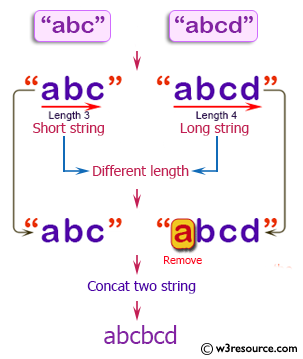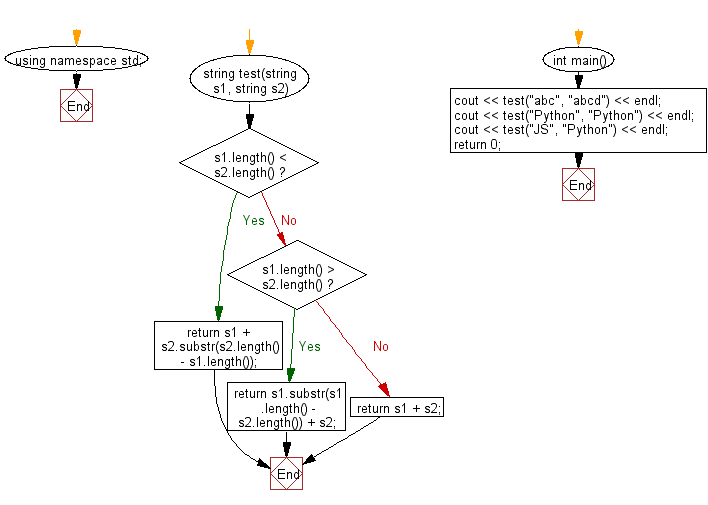﻿ C++ Exercises: Concat two given strings - w3resource# C++ Exercises: Concat two given strings

## C++ Basic Algorithm: Exercise-76 with Solution

Write a C++ program to concat two given strings. If the given strings have different length remove the characters from the longer string.

Sample Solution:

C++ Code :

``````#include <iostream>
using namespace std;

string test(string s1, string s2)
{
if (s1.length() < s2.length())
{
return s1 + s2.substr(s2.length() - s1.length());
}
else if (s1.length() > s2.length())
{
return s1.substr(s1.length() - s2.length()) + s2;
}
else
{
return s1 + s2;
}
}

int main()
{
cout << test("abc", "abcd") << endl;
cout << test("Python", "Python") << endl;
cout << test("JS", "Python") << endl;
return 0;
}
``````

Sample Output:

```abcbcd
PythonPython
JSon
```

Pictorial Presentation:Flowchart:C++ Code Editor: